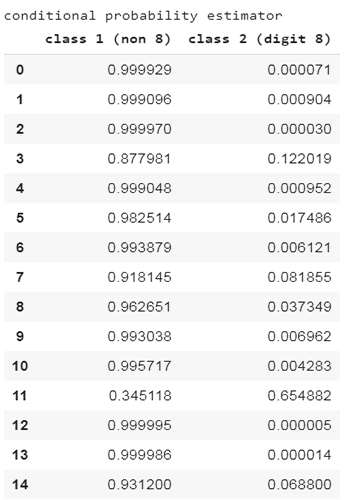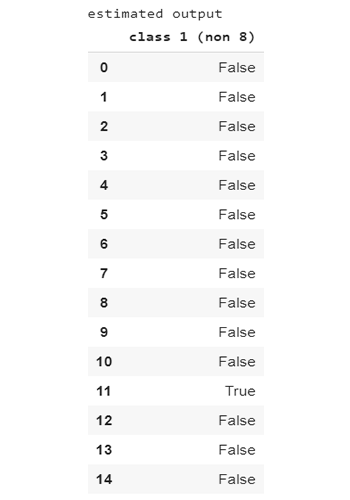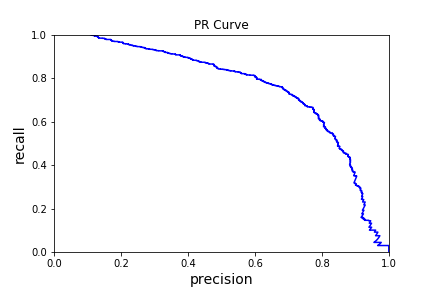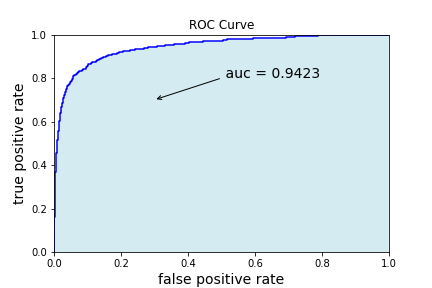New offer! Get 30% off your first 2 months of Unlimited Monthly. Start your subscription for just £29.99 £19.99. New subscribers only. T&Cs apply

•# How to assess the goodness of fit in classification?

In this article, we introduce several standard test metrics to evaluate the model performance in the classification tasks.

For classification tasks, the accuracy and confusion matrix are the most popular metrics to quantify the goodness of fit. For the binary classification, one may consider other evaluation methods, e.g., precision, recall, PR curve and ROC curve, which are particularly useful for the imbalance problem.

Let us first recall how to make the model prediction and then introduce the test metrics mentioned above for the classification tasks.

## Model prediction

Let (f_{theta^{*}}) denote the trained classifier, where (theta^{*}) is the optimal model parameters. The prediction is straightforward; for any new input data (x_{*}), we use the output label with the highest estimated conditional probability as the output estimator, i.e.,

[hat{y}_{*} = text{arg}max_{i in mathcal{Y}} f_{theta_{*}}^{(i)}(x_{*}),]

where (f_{theta_{*}}^{(i)}(x_{*})) is the (i^{th}) coordinate of (f_{theta_{*}}(x_{*})).

## Model evaluation

At the final stage, we need to specify the metric for the goodness of fit. There are various performance measures, e.g. the accuracy, the confusion matrix, etc.

### Accuracy

In classification, the dimension of the model output (f_{theta^{*}}(x)) is (n_o), which represents the estimated conditional probability of each output. Let (hat{Y}_{prob}) denote the matrix of size ((N, n_{o})),

[hat{Y}_{text{prob}} = (f_{theta^{*}}(x_{i}))_{iin {1, 2, cdots, N}}.]

For the multi-class classification, accuracy is one of the most popular measures and is defined as follows:

[sum_{i = 1}^{N}frac{mathbf{1}(hat{y}_{i} = y_{i})}{N},]

where (i in {1, 2, cdots, N}), (y_{i}) and (hat{y}_{i}) denote the actual output and the estimated output of the (i^{th}) sample respectively.

### Confusion matrix

Another way to measure the performance of the classifier is the confusion matrix. The column represents the estimated label for the classification problem, and the row represents the true label. Let (M:= (M_{i, j})_{i, j in mathcal{Y}}) denote the confusion matrix, where (M_{i,j}) denotes the number of samples with true label (i) and the estimated label (j). The better the prediction is, the more diagonally dominant the confusion matrix (M) is.

The normalized confusion matrix is defined on top of the confusion matrix, denoted by (hat{M} = (hat{M}_{i, j})_{i, j in mathcal{Y}}), where (hat{M}_{i, j}) is defined as follows:

[hat{M}_{i, j} = frac{M_{i, j}}{sum_{j in mathcal{Y}}M_{i, j}}.]

Here (hat{M}_{i, j}) represents the empirical conditional probability of the sample being identified (j) on the condition that the sample belongs to class (i). The better the prediction is, the closer to the identity matrix (hat{M}) is.

### Other metrics for binary classification

You may wonder why we need other metrics rather than accuracy to assess the classification performance. When the data is extremely imbalanced, the trivial classifier (estimating all samples as the majority class) gives very high accuracy, which implies that the accuracy is not an informative performance measurement in this case. Next, we introduce some other commonly used metrics for the binary classification case, e.g., precision, recall, PR curve, ROC curve etc.

As shown in the figure below, the confusion matrix of a binary classifier (M=(M_{j_{1}, j_{2}})_{j_{1}, j_{2} in { 1, 2}}) is a (2 times 2) matrix, where

• True Positive (TP, (M_{2,2})): the number of the samples which have actual label being class (2) and predicted label being class (2);
• False Positive (FP, (M_{1,2})): the number of the samples which have actual label being class (1) and predicted label being class (2);
• True Negative (TN, (M_{1,1})): the number of the samples which have actual label being class (1) and predicted label being class (1);
• False Negative (FN, (M_{2,1})): the number of the samples which have actual label being class (2) and predicted label being class (1).The precision of a binary classifier is defined as the percentage of true positive samples among all the samples with the predicted label being “positive”:

[text{precision} = frac{text{TP}}{text{TP}+text{FP}}.]

The recall is also called the sensitivity or true positive ratio (TPR) and defined as the percentage of true positive samples among all the samples with actual label being “positive”:

[text{recall} = frac{text{TP}}{text{TP}+text{FN}}.]

From the above definition, we can see that one trivial way to get a high recall is to predict all the samples being “positive”, which gives the perfect recall (100%). Thus recall is typically used accompanied by precision. The higher recall suggests a larger TP, which indicates the better performance of a classifier. But increasing the recall reduces the precision and vice versa. This is called the precision and recall trade-off.

Next, let us introduce the Precision-Recall curve (PR curve) based on the above concepts of precision and recall. For a classifier, (f_{theta^{*}}) gives an estimated probability (also called a score) to each possible output class. Instead of choosing the class label which gives the maximum score, alternatively, for each given threshold value (t), we assign the estimator for the output using the following equation:

[hat{y} = begin{cases} 1, &text{if } f_{theta^{*}}(x) > t;\ 2, &text{if } f_{theta^{*}}(x) leq t. end{cases}]

Varying the threshold (t), the corresponding precision and recall can be computed, and thus the PR curve is obtained.

The Receiver Operating Characteristic curve (ROC curve) is another important metric of a binary classifier. Similar to the PR curve, varying the threshold (t), the ROC curve is the curve of TP against the FP. AUC stands for “Area under the ROC Curve’’. It measures the entire two-dimensional area enclosed by the ROC curve, a cord from ((0,0)) to ((1,0)) and a cord from ((1, 0)) and ((1, 1)).

## A numerical example

In the following, we use the binary classification of identifying whether a digit image is a number (8) as a concrete example to show how to compute all metrics we have discussed and implemented it using Scikit-Learn.

We use the MNIST dataset (http://yann.lecun.com/exdb/mnist/) composed of digit images of Numbers 0-9. The input data is a grey-valued image and the output is the digit in the input image. Now we want to identify whether an input image is a digit (8) and construct a binary classifier where class (1) represents “non-(8) digit” while class (2) represents “digit (8)”. The training dataset contains (60,000) handwritten digit image, including (54,149) non-(8) digit samples and (5,851) digit (8) samples. It is easy to see that the number of negative class labels is much more than that of the positive ones. Thus it is a class imbalance problem. In the left panel of the following figure, each row shows the probabilities of each sample belonging to class 1 or class 2, summing up to (1). The estimated class label is the label with the maximum probability, shown in the right panel.

Conditional probability Estimated outputWe first compute the confusion matrix using confusion_matrix() in scikit-learn package as shown below:

from sklearn.metrics import confusion_matrix # Y_train is a binary vector of the actual class label with dim (N, 1) where N is the number of samples;# y_train_est is a binary vector of the estimated class label with dim (N, 1). cm = confusion_matrix(Y_train, y_train_est)print('confusion matrix is {}'.format(cm))

Screen output

The confusion matrix is

[left(begin{matrix}
53073 & 1076\
1885 &3966
end{matrix}right).]

Based on the confusion matrix, we can compute the corresponding accuracy via

[text{accuracy} = frac{text{TP}+text{TN}}{text{TP}+text{FN}+text{TN}+text{FP}} = frac{53073+3966}{53073+1076+ 1885 + 3966} = 0.95065.]

You may also use accuracy_score() in sklearn.metrics to compute the accuracy of (hat{Y}).

from sklearn.metrics import accuracy_scoreacc = accuracy_score(Y_train, y_train_est)

The accuracy is about (95%), which seems very good. But if one compute the precision and recall, the prediction is not that great.

[text{precision} = frac{text{TP}}{text{TP}+text{FP}} = frac{3966}{3966+1076} = 0.786592]

[text{recall} = frac{text{TP}}{text{TP}+text{FN}} = frac{3966}{3966+1885} = 0.677833]

Similar to the accuracy case, you may use the following Python function to compute the precision and recall.

from sklearn.metrics import precision_score, recall_scoreprecision = precision_score(Y_train, y_train_est)recall = recall_score(Y_train, y_train_est)

The following two figures provide the codes for computing the PR curve and ROC curve obtained by a binary classification task using scikit-learn Python package. In this example, the AUC score is (0.9431), which is the area of the blue-shaded region enclosed under the ROC curve.

from sklearn.metrics import precision_recall_curve precisions, recalls, thresholds = precision_recall_curve(Y_train, y_train_prob_est[:,1])plt.plot(precisions, recalls, 'b')plt.xlabel('precision', fontsize=14)plt.ylabel('recall', fontsize=14)plt.title('PR Curve')plt.axis([0, 1, 0, 1])

Screen outputfrom sklearn.metrics import roc_curve, roc_auc_scorefps, tps, thresholds = roc_curve(Y_train, y_train_prob_est[:,1])roc_auc_score_train = roc_auc_score(Y_train, y_train_prob_est[:,1])import matplotlib.pyplot as pltplt.plot(fps, tps, 'b')plt.xlabel('false positive rate', fontsize=14)plt.ylabel('true positive rate', fontsize=14)plt.title('ROC Curve')plt.axis([0, 1, 0, 1])plt.fill_between(fps, 0, tps, facecolor='lightblue', alpha=0.5)plt.text( 0.5, 0.8, 'roc auc score = '+str(round(roc_auc_score_train, 4)), fontsize=14)plt.annotate("", xy=(0.3, 0.7), xycoords='data', xytext=(0.5, 0.8), textcoords='data', arrowprops=dict(arrowstyle="->", connectionstyle="arc3"),)When the positive class has much fewer samples than the negative class and the false positives are more important, one should choose the PR curve. For example, looking at the previous ROC curve and the AUC score, you may think that the classifier is really good. But this is mostly because there are few positives compared to the negatives.

## Summary

In conclusion, we provide a summary of the general framework of the classification.

• Dataset: (mathcal{D} = {(x_{i}, y_{i})}_{i = 1}^{N}).
• Model: (f_{theta}(x, y) approx P(y vert x)) , (forall x in mathbb{R}^{d}), (y in mathcal{Y}).
• Empirical Loss: (L(theta vert mathcal{D}) = – frac{1}{N}underset{i = 1}{overset{N}{sum}}log (f_{theta}(x_{i}, y_{i})) rightarrow min).
• Optimization: (theta^{*} = underset{theta}{argmin} (L(theta vert mathcal{D}))).
• Prediction: (hat{y}_{*} = underset{y in mathcal{Y}}{text{arg} max}f_{theta^{*}}(x_{*}, y)).
• Validation: Accuracy, confusion matrix, etc.